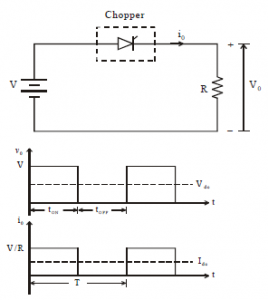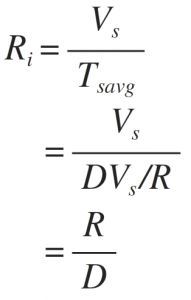Home > dc dc converter chopper > step down chopper

# Step Down Chopper

Step down chopper for R load

Figure below shows the Circuit Diagram andÂ  Waveform of resistive load step down chopper.â€¢ The thyristor in the circuit acts as a switch.
â€¢ When thyristor is ON, supply voltage appears across the load
â€¢ When thyristor is OFF, the voltage across the load will be zero.

The average output voltage is given as,

Where, D is duty cycle = TON/T.
TON can be varied from 0 to T, so 0 â‰¤ D â‰¤ 1.

Hence output voltage VO can be varied from0 to VS.

Therefore effective input resistance is given by,so, we can conclude that output voltage is always less than the input voltage and hence the name step down chopper is justified. The output voltage and current waveform of step down chopper with resistive load is shown in above figure.